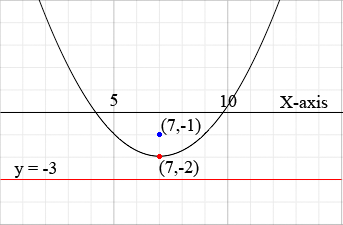SEARCH HOMEMath Central Quandaries & QueriesQuestion from Deann, a student: Find an equation of the parabola with vertex (7,-2) and directrix y =(-3)Hi Deann,

Since the directrix is y = -3 and the vertex is (7,-2) you can draw a rough sketch.The distance from the vertex to the directrix is 1 unit. Since every point on a parabola is the same distance from the directrix as it is from the focus the focus must be at (7,-1). Can you write the equation of the parabola with directrix y = -3 and focus (7,-1)?

PennyMath Central is supported by the University of Regina and The Pacific Institute for the Mathematical Sciences.